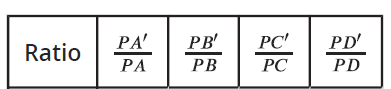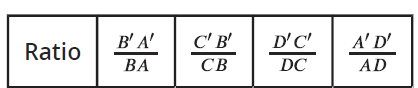# IM Geometry Unit 3 Lesson 3

Topic:
Geometry

## Dilate triangle FGH using center C and a scale factor of 3.

Below is triangle EFG and center C. What are the lengths of each side of the image of triangle EFG if you dilate triangle EFG by a scale factor of 3?

Do the following dilations on triangle EFG below:
• Dilate triangle EFG by a scale factor of 3 using center C.
• Dilate triangle EFG by a scale factor of 1/2 using center C.
• Dilate triangle EFG by a scale factor of 2 using center C.

## Below is a dilation of parallelogram ABCD by a scale factor of 1/2 using center E.

Find each of the following ratios:What do you notice?

## Below is a dilation of parallelogram ABCD by a scale factor of 1/2 using center E.

Find each of the following ratios:What do you notice?